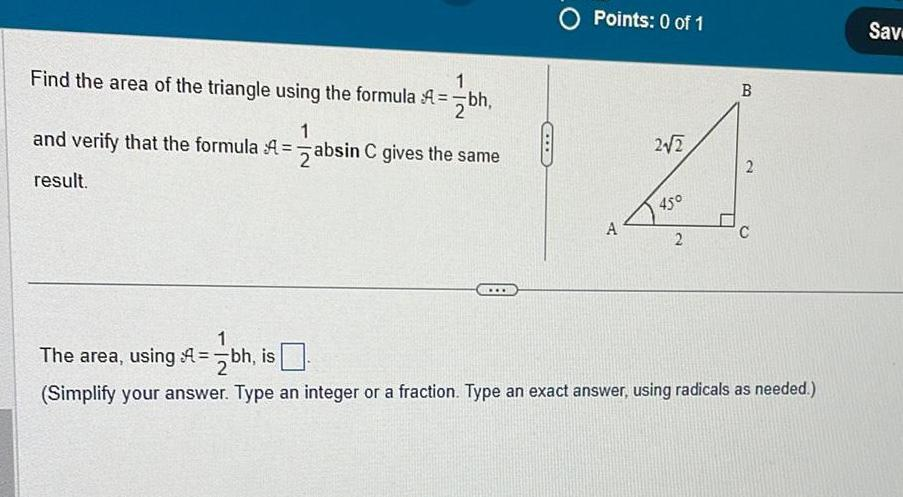Question:

# Find the area of the triangle using the formula A bh 1 and

Last updated: 11/20/2023Find the area of the triangle using the formula A bh 1 and verify that the formula A absin C gives the same result O Points 0 of 1 A 2 2 45 2 B 2 1 The area using bh is Simplify your answer Type an integer or a fraction Type an exact answer using radicals as needed Sav